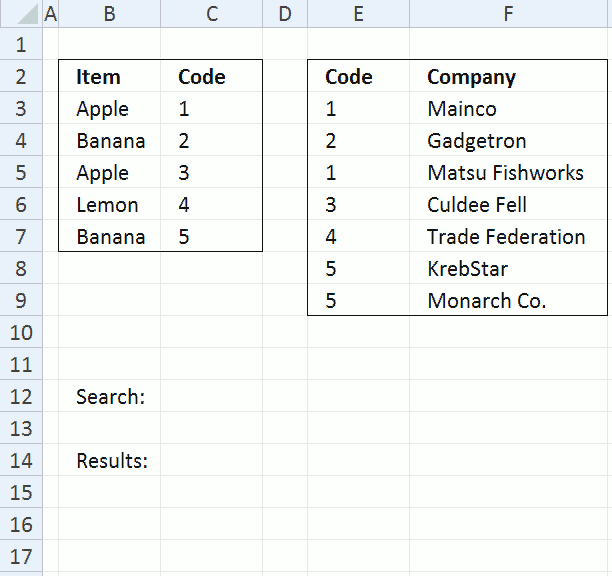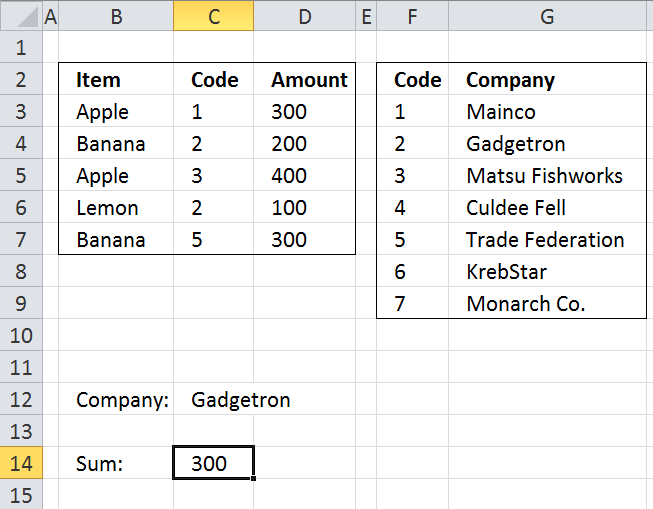Author: Oscar Cronquist Article last updated on July 29, 2017

The "new" excel 2010 powerpivot feature and DAX formulas lets you work with multiple tables of data. You can connect tables to each other by relationships. When relationships are made nothing stops you from doing lookups to related values and related tables or sum values for a related table.

This post is not about powerpivot and DAX formulas, it is about doing lookups in two tables and they have one column in common. I´ll also show you how to sum values in a related table.

### Search for values in a related tableArray formula in cell C14:

=IFERROR(INDEX(\$F\$3:\$F\$9, SMALL(IF(ISERROR(MATCH(\$E\$3:\$E\$9, IF(\$C\$12=\$B\$3:\$B\$7, \$C\$3:\$C\$7, ""), 0)), "", MATCH(ROW(\$F\$3:\$F\$9), ROW(\$F\$3:\$F\$9))), ROW(A1))), "")

How to create an array formula

1. Select cell C14
2. Copy above array formula
3. Click in formula bar
4. Paste array formula
5. Press and hold Ctrl + Shift
6. Press Enter

How to copy array formula

1. Select cell C14
2. Copy (Ctrl + c)
3. Select cell range C15:C17
4. Paste (Ctrl + v)

Explaining array formula in cell C14

Step 1 - Search for a value

IF(\$C\$12=\$B\$3:\$B\$7, \$C\$3:\$C\$7, "")

becomes

IF("Apple"={"Apple"; "Banana"; "Apple"; "Lemon"; "Banana"}, {1; 2; 3; 4; 5}, "")

and returns {1;"";3;"";""}.

Step 2 - Use column in common to find matches

MATCH(\$E\$3:\$E\$9, IF(\$C\$12=\$B\$3:\$B\$7, \$C\$3:\$C\$7, ""), 0)

becomes

MATCH(\$E\$3:\$E\$9, {1;"";3;"";""}, 0)

becomes

MATCH({1; 2; 1; 3; 4; 5; 5}, {1;"";3;"";""}, 0)

and returns

{1;#N/A;1;3;#N/A;#N/A;#N/A}

Step 3 - Return row numbers

IF(ISERROR(MATCH(\$E\$3:\$E\$9, IF(\$C\$12=\$B\$3:\$B\$7, \$C\$3:\$C\$7, ""), 0)), "", MATCH(ROW(\$F\$3:\$F\$9), ROW(\$F\$3:\$F\$9)))

becomes

IF(ISERROR({1;#N/A;1;3;#N/A;#N/A;#N/A}, "", MATCH(ROW(\$F\$3:\$F\$9), ROW(\$F\$3:\$F\$9)))

becomes

IF(ISERROR({1;#N/A;1;3;#N/A;#N/A;#N/A}, "", {1; 2; 3; 4; 5; 6; 7})

and returns {1; ""; 3; 4; ""; ""; ""}

Step 4 - Return a value of the cell at the intersection of  a particular row and column

=INDEX(\$F\$3:\$F\$9, SMALL(IF(ISERROR(MATCH(\$E\$3:\$E\$9, IF(\$C\$12=\$B\$3:\$B\$7, \$C\$3:\$C\$7, ""), 0)), "", MATCH(ROW(\$F\$3:\$F\$9), ROW(\$F\$3:\$F\$9))), ROW(A1)))

becomes

=INDEX(\$F\$3:\$F\$9, SMALL({1; ""; 3; 4; ""; ""; ""}, ROW(A1)))

becomes

=INDEX(\$F\$3:\$F\$9, 1)

becomes

=INDEX({"Mainco"; "Gadgetron"; "Matsu Fishworks"; "Culdee Fell"; "Trade Federation"; "KrebStar"; "Monarch Co."}, 1)

and returns Mainco in cell C14.

### Sum values in a related tableArray formula in cell C14:

=SUM(IF(ISERROR(MATCH(\$C\$3:\$C\$7, IF(\$C\$12=\$G\$3:\$G\$9, \$F\$3:\$F\$9, ""), 0)), 0, \$D\$3:\$D\$7))

How to create an array formula